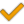## You are here

#### Mixtures according to GERG-2008

The GERG-2008 equation is valid in the gas phase, in the liquid phase, in the supercritical region and for the vapor-liquid equilibrium (VLE). The entire range of validity regarding the calculation of thermodynamic properties of natural gases, other multi-component mixtures and binary mixtures is divided, as follows, into three different parts. The uncertainty estimations given for the different ranges of validity are based on the representation of experimental data of various thermodynamic properties of binary and multi-component mixtures by the GERG-2008 reference equation of state.

The available components you find below.

The following components are available for AGA 8 and GERG 2008:

Component GERG 2008 AGA 8
MethaneNitrogenCarbon dioxideEthanePropanen-Butanei-Butanen-Pentanei-Pentanen-Hexanen-Heptanen-Octanen-Nonanen-DecaneHydrogenOyxgenCarbon monoxideWaterHydrogen sulphurHeliumArgonNormal range of validity

The normal range of validity covers temperatures of 90 K ≤ T ≤ 450 K and pressures of p ≤ 35 MPa.

This range corresponds to the use of the equation in both standard and advanced technical applications using natural gases, e.g. pipeline transport, natural gas storage and processes with liquefied natural gas.

The uncertainty of the equation regarding gas phase density and speed of sound is less than 0.1% in the temperature range from 250 K/270 K to 450 K at pressures up to 35 MPa. This uncertainty is valid for various types of natural gases as well as for many binary and other mixtures consisting of the 18 natural gas components covered by GERG-2008. Furthermore, the GERG-2008 equation represents accurate data of isobaric enthalpy differences of binary and multi-component mixtures to within their experimental uncertainty, which is less than (0.2 – 0.5)%.

In the liquid phase of many binary and multi-component mixtures the uncertainty of the equation with regard to density amounts to approximately less than (0.1 – 0.5)%.

Due to restrictions from the poor data situation, the vapor-liquid phase equilibrium is described with reasonable accuracy. Hence, accurate vapor pressure data of binary and ternary mixtures consisting of the natural gas main components are reproduced by the equation to within their experimental uncertainty, which is approximately (1 – 3)%.

Extended range of validity

The extended range of validity covers temperatures of 60 K ≤ T ≤ 700 K and pressures of p ≤ 70 MPa.

The uncertainty of the equation regarding gas phase density at temperatures and pressures outside the normal range of validity is roughly estimated to be less than (0.2 – 0.5)%.

For certain mixtures, the extended range of validity covers temperatures up to 900 K and pressures up to 100 MPa and more. For example, the equation accurately describes gas phase density data of air within ±(0.1 – 0.2)% at temperatures up to 900 K and pressures up to 100 MPa.

The calculation of properties beyond the extended range of validity

When accepting larger uncertainties, the equation can be reasonably used outside the extended range of validity. For example, data of certain binary mixtures are described within ±1% at pressures up to 300 MPa.